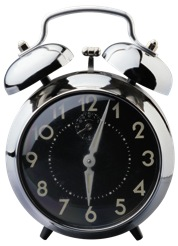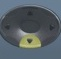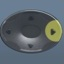## TimeBasics

• In time-related questions, you will need to calculate how much time has passed.
• In many of these questions, you will need to break up time and add the sum of its parts.
• To answer a question involving different time zones, you will need to adjust the departure time to coincide with the arrival time zone.
• The on-screen calculator may or may not come into play with these questions.Question

How much time has passed between 9:45 AM and 4:15 PM?  Express your answer in hours and minutes.

6 hours 30 minutes

9:45 AM – 10:00 AM = 15 minutes
10:00 AM – 12:00 PM (Noon) = 2 hours
12:00 PM – 4:00 PM = 4 hours
4:00 PM – 4:15 PM = 15 minutes

Time = 15 minutes + 2 hours + 4 hours + 15 minutes = 6 hours 30 minutes

Question
With reference to the question above, express your answer in hours as a mixed number.$\bf\displaystyle6\frac{1}{2}$ hours

9:45 AM – 10:00 AM = 15 minutes
10:00 AM – 12:00 PM (Noon) = 2 hours
12:00 PM – 4:00 PM = 4 hours
4:00 PM – 4:15 PM = 15 minutes

Time = 15 minutes + 2 hours + 4 hours + 15 minutes = 6 hours 30 minutes
Convert 30 minutes to a fraction of an hour:$\bf\displaystyle\frac{30\,minutes}{60\,minutes\,per\,hour}$ =$\bf\displaystyle\frac{1}{2}$ hour

Time =$\bf\displaystyle6\frac{1}{2}$ hours

Calculator Click What You See Commentblinker clears screen3060$\bf\displaystyle\frac{30}{60}$ ratio of 30 minutes to
60 minutes per hour$\bf\displaystyle\frac{1}{2}$ 30 minutes converted to$\bf\displaystyle\frac{1}{2}$ hour
###### Time

Question

With reference to the question above, express your answer in hours in decimal form.

6.5 hours

9:45 AM – 10:00 AM = 15 minutes
10:00 AM – 12:00 PM (Noon) = 2 hours
12:00 PM – 4:00 PM = 4 hours
4:00 PM – 4:15 PM = 15 minutes

Time = 15 minutes + 2 hours + 4 hours + 15 minutes = 6 hours 30 minutes
Convert 30 minutes to a fraction of an hour:$\bf\displaystyle\frac{30\,minutes}{60\,minutes\,per\,hour}$ =$\bf\displaystyle\frac{1}{2}$ hour
Convert$\bf\displaystyle\frac{1}{2}$ hour to a decimal:$\bf\displaystyle\frac{1}{2}$ hour = 1 ÷ 2 = 0.5 hour

Time = 6.5 hours

Calculator Click What You See Commentblinker clears screen3060$\bf\displaystyle\frac{30}{60}$ ratio of 30 minutes to
60 minutes per hour$\bf\displaystyle\frac{1}{2}$ 30 minutes converted to$\bf\displaystyle\frac{1}{2}$ hour0.5$\bf\displaystyle\frac{1}{2}$ hour converted to 0.5 hour
(Toggle)
###### Time

Question
How much time has passed between 9:15 AM and 4:45 PM?  Express your answer in hours and minutes.

7 hours 30 minutes

9:15 AM – 10:00 AM = 45 minutes
10:00 AM – 12:00 PM (Noon) = 2 hours
12:00 PM – 4:00 PM = 4 hours
4:00 PM – 4:45 PM = 45 minutes

Time = 45 minutes + 2 hours + 4 hours + 45 minutes =
6 hours 90 minutes = 7 hours 30 minutes

Question
A plane departs from New York City at 8:00 AM Eastern Standard Time (EST) and arrives in Los Angeles at 11:00 AM Pacific Standard Time (PST).  How many hours did the flight take?

6 hours

Adjust the departure time to coincide with the arrival time zone.

Departure: 8:00 AM EST = 5:00 AM PST
Arrival: 11:00 AM PST
5:00 AM PST —> 11:00 AM PST = 6 hours

Question

A plane departs from Chicago at 6:00 AM Central Standard Time (CST) and arrives in Miami at 10:00 AM Eastern Standard Time (EST).  How many hours did the flight take?

3 hours

Adjust the departure time to coincide with the arrival time zone.

Departure: 6:00 AM CST = 7:00 AM EST
Arrival: 10:00 AM EST
7:00 AM EST —> 10:00 AM EST = 3 hours

Practice – Questions
1.  How much time has passed between 7:45 AM and 3:30 PM?  Express your answer in hours and minutes.

2.  With reference to Question 1, express your answer in hours as a mixed number.

3.  With reference to Question 1, express your answer in hours in decimal form.

4.  How much time has passed between 7:45 AM and 3:55 PM?  Express your answer in hours and minutes.

5.  How much time has passed between 5:45 PM and 2:30 AM?  Express your answer in hours and minutes.

6.  How much time has passed between 5:15 PM and 2:55 AM?  Express your answer in hours and minutes.

7.  A plane departs from New York City at 7:00 AM Eastern Standard Time (EST) and arrives in Los Angeles at 10:00 AM Pacific Standard Time (PST).  How many hours did the flight take?

8.  A jet departs from New York City at 1:00 PM Eastern Standard Time (EST) and arrives in Los Angeles at 11:00 AM Pacific Standard Time (PST).  How many hours did the flight take?

9.  A plane departs from Denver at 10:00 AM Mountain Standard Time (MST) and arrives in Boston at 4:00 PM Eastern Standard Time (EST).  How many hours did the flight take?

10.  A jet departs from Denver at 10:00 AM Mountain Standard Time (MST) and arrives in Boston at 1:00 PM Eastern Standard Time (EST).  How many hours did the flight take?

1.  7 hours 45 minutes

2.$\bf\displaystyle7\frac{3}{4}$ hours

3.  7.75 hours

4.  8 hours 10 minutes

5.  8 hours 45 minutes

6.  9 hours 40 minutes

7.  6 hours

8.  1 hour

9.  4 hours

10.  1 hour